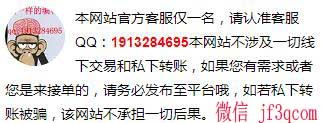# c++求连续子数组的最大和

2021-01-06 23:00:09   223浏览 分类: Java

`/* * 求子数组得最大和 - C++ - by www.jf3q.com * * 题目：输入一个整形数组，数组里有正数也有负数。数组中连续得一个或多个整数组成一个子数组，每个子数组都有一个和。 * 求所有子数组得和得最大值。要求时间复杂度为O(n)。 * 例如输入得数组为5, -22, 13, 0, 45, -7, 42, -19；和最大得子数组为13, 0, 45, -7, 42；因此输出为该子数组得和为93。 * * Answer: A traditional greedy approach. */ #include <iostream>#include <stdio.h> using namespace std; int MaxSum(int *a, const int size) {    if (size <= 0) {        length_error("Error, wrong array size.");    }     int sum = 0;    int max = -(1 << 31);    int cur = 0;     while (cur < size) {        sum += a[cur++];         if (sum > max) {            max = sum;        }         if (sum < 0) {            sum = 0;        }    }     return max;} int main(int argc, char *argv[]) {    int a[] = {5, -22, 13, 0, 45, -7, 42, -19};    cout << MaxSum(a, 8) << endl;     return 0;} // Output:`
/*

*/

##### 公告##### 第一时间了解动态##### 关注我们